• Ensemble Kalman filter集合卡尔曼滤波
万次阅读 多人点赞
2021-02-03 14:28:12

在气象预测领域，很多时候，模型具有 O ( 10 e 8 ) O(10e8) 以上的量级，如果使用传统的卡尔曼滤波，协方差矩阵的更新将是一个~ 10 e 22 10e22 量级的计算操作，因此传统的卡尔曼滤波并不适用。集合卡尔曼滤波（Ensemble Kalman filter）用sample covariance模拟原本的协方差矩阵，用一个小的多的量级的矩阵运算代替原本的协方差矩阵更新。集合卡尔曼滤波与粒子滤波有相通之处，集合卡尔曼滤波假设用来做模拟的ensembles符合高斯分布。

本文将通过一个应用EnKF进行定位的python实例来初探集合卡尔曼滤波的概念。

根据参考【1】，集合卡尔曼滤波的公式为：用通俗的话来解释参考【2】例子中的EnKF：对于机器人状态向量 X = [ x , y , θ , v ] X=[x, y, \theta,v] ，生成数量为N的ensembles，每一个ensemble都是机器人状态向量加上一个方差为 Q Q 的误差，如此一来，通过上面的(12)(13)式我们可以看出，状态向量协方差矩阵 P P 已经不需要显式地计算出来了，我们只要针对每一个ensemble做计算就好了，而在现实问题中，ensemble的数量要远小于 P P 的维度，这就是集合卡尔曼滤波的精髓。

下面我们来看参考【2】中的代码：

for ip in range(NP):
x = np.array([px[:, ip]]).T
#  Predict with random input sampling
# 预测：给输入加上方差为Q的随机误差：
ud1 = u[0, 0] + np.random.randn() * Q[0, 0] ** 0.5
ud2 = u[1, 0] + np.random.randn() * Q[1, 1] ** 0.5
ud = np.array([[ud1, ud2]]).T
x = motion_model(x, ud)
px[:, ip] = x[:, 0]


对应公式(10)，给运动模型的输入加上 q i q_i ，计算每一个ensemble的状态向量的值。

接下来：

        # 根据状态预测值x和观测值z获取路标点加上观测方差R的空间位置z_pos
z_pos = observe_landmark_position(x, z)
pz[:, ip] = z_pos[:, 0]


在这里我认为observe_landmark_position函数计算的其实是：

H x i f + r i Hx_i^f+r_i

原因在计算(17)时会提到。

    x_ave = np.mean(px, axis=1)
# 计算 x_f-x_f_bar
x_dif = px - np.tile(x_ave, (NP, 1)).T

z_ave = np.mean(pz, axis=1)
# 计算 Hx_f-Hx_f_bar
z_dif = pz - np.tile(z_ave, (NP, 1)).T

U = 1 / (NP - 1) * x_dif @ z_dif.T
V = 1 / (NP - 1) * z_dif @ z_dif.T


接下来 U U V V 我认为分别对应(12)(13)。在计算卡尔曼增益 K K 时，原代码直接计算：

K = U @ np.linalg.inv(V)


不知为什么好像没有体现出(14)式中的观测协方差 R R ，难道是因为我在上面提到的 p z pz 中已经包含了观测噪声 r r

我对原代码进行了一些改动，加入了观测方差 R R （不能保证理论上的正确性），改动后的定位效果目测与原先没什么区别。

    # 以下是我的改动：
# 观测协方差矩阵：
R_a = np.diag([R[0,0]/2, R[0,0]/2] * z.shape)
# 原代码：K = U @ np.linalg.inv(V)分母不包含观测协方差矩阵，
# 这似乎与参考论文所述K的计算方法不符，不知道为什么这样处理
# 在加入观测协方差R计算K后，与不加R计算K在定位结果上**目测**没有什么差别
K = U @ np.linalg.inv(V + R_a)  # Kalman Gain


最后计算残差并进行更新：

    # 获取观测的真值：
z_lm_pos = z[:, [2, 3]].reshape(-1, )

# 更新：在这里pz包含了Hx_f和观测噪声r
px_hat = px + K @ (np.tile(z_lm_pos, (NP, 1)).T - pz)


在这里我们看到更新时直接用路标点真值减去了 p z pz ，这也是为什么上面我提到observe_landmark_position函数计算的其实是 H x i f + r i Hx_i^f+r_i

完整的包含注释的python代码如下：

"""

Ensemble Kalman Filter(EnKF) localization sample

author: Ryohei Sasaki(rsasaki0109)

modification: Junchuan Zhang

Ref:
Ensemble Kalman filtering
(https://rmets.onlinelibrary.wiley.com/doi/10.1256/qj.05.135)

"""

import math

import matplotlib.pyplot as plt
import numpy as np
from scipy.spatial.transform import Rotation as Rot

# 为了代码阅读上的方便，我在此加入了区别于simulation parameter的Q和R；
# Q和R数值上与simulation parameter一致（只是为了简化计算）
# 运动模型输入协方差：
Q = np.diag([1.0,np.deg2rad(30.0)]) ** 2  # predict state covariance
# 观测模型协方差：
R = np.diag([0.2, np.deg2rad(1.0)]) ** 2  # Observation covariance

#  Simulation parameter
# 输入：
Q_sim = np.diag([1.0, np.deg2rad(30.0)]) ** 2
# 观测：
R_sim = np.diag([0.2, np.deg2rad(1.0)]) ** 2

DT = 0.1  # time tick [s]
SIM_TIME = 50  # simulation time [s]
MAX_RANGE = 20.0  # maximum observation range

# Ensemble Kalman filter parameter
# 很显然，增大NP，精度提高，计算量也随之提高
NP = 20  # Number of Particle

show_animation = True

def calc_input():
v = 1.0  # [m/s]
u = np.array([[v, yaw_rate]]).T
return u

def observation(xTrue, xd, u, RFID):
xTrue = motion_model(xTrue, u)

z = np.zeros((0, 4))

for i in range(len(RFID[:, 0])):

dx = RFID[i, 0] - xTrue[0, 0]
dy = RFID[i, 1] - xTrue[1, 0]
d = math.hypot(dx, dy)
angle = pi_2_pi(math.atan2(dy, dx) - xTrue[2, 0])
if d <= MAX_RANGE:
dn = d + np.random.randn() * R_sim[0, 0] ** 0.5  # add noise
angle_with_noise = angle + np.random.randn() * R_sim[1, 1] ** 0.5
# 在此将RFID的位置真值也一起放入了每组观测值内：
zi = np.array([dn, angle_with_noise, RFID[i, 0], RFID[i, 1]])
z = np.vstack((z, zi))

ud = np.array([[
u[0, 0] + np.random.randn() * Q_sim[0, 0] ** 0.5,
u[1, 0] + np.random.randn() * Q_sim[1, 1] ** 0.5]]).T

xd = motion_model(xd, ud)
return xTrue, z, xd, ud

def motion_model(x, u):
F = np.array([[1.0, 0, 0, 0],
[0, 1.0, 0, 0],
[0, 0, 1.0, 0],
[0, 0, 0, 0]])

B = np.array([[DT * math.cos(x[2, 0]), 0],
[DT * math.sin(x[2, 0]), 0],
[0.0, DT],
[1.0, 0.0]])
x = F.dot(x) + B.dot(u)

return x

def observe_landmark_position(x, landmarks):
'''
根据机器人空间位置x和路标点的观测值landmarks，
计算加入观测噪声后的路标点空间值
'''
# landmark_pos大小为2倍路标点个数，因为将路标点x和y位置都放在了一个维度上：
landmarks_pos = np.zeros((2 * landmarks.shape, 1))
for (i, lm) in enumerate(landmarks):
index = 2 * i
# 在这里只包含R[0,0]，因为路标点x和y只涉及距离误差：
q = R[0, 0] ** 0.5
# 因此每一维加上sqrt(距离协方差/2)：
landmarks_pos[index] = x[0, 0] + lm * math.cos(
x[2, 0] + lm) + np.random.randn() * q / np.sqrt(2)
landmarks_pos[index + 1] = x[1, 0] + lm * math.sin(
x[2, 0] + lm) + np.random.randn() * q / np.sqrt(2)
return landmarks_pos

def calc_covariance(xEst, px):
'''
计算滤波更新后状态集合的方差
'''
cov = np.zeros((3, 3))

for i in range(px.shape):
dx = (px[:, i] - xEst)[0:3]
cov += dx.dot(dx.T)
cov /= NP

return cov

def enkf_localization(px, z, u):
"""
Localization with Ensemble Kalman filter
z: 前两位是观测，后两位是RFID的值
u: 含有噪声的输入
"""
# print("________")
pz = np.zeros((z.shape * 2, NP))  # Particle store of z
for ip in range(NP):
x = np.array([px[:, ip]]).T
#  Predict with random input sampling
# 预测：给输入加上方差为Q的随机误差：
ud1 = u[0, 0] + np.random.randn() * Q[0, 0] ** 0.5
ud2 = u[1, 0] + np.random.randn() * Q[1, 1] ** 0.5
ud = np.array([[ud1, ud2]]).T
x = motion_model(x, ud)
px[:, ip] = x[:, 0]
# 根据状态预测值x和观测值z获取路标点加上观测方差R的空间位置z_pos
z_pos = observe_landmark_position(x, z)
pz[:, ip] = z_pos[:, 0]

x_ave = np.mean(px, axis=1)
# 计算 x_f-x_f_bar
x_dif = px - np.tile(x_ave, (NP, 1)).T

z_ave = np.mean(pz, axis=1)
# 计算 Hx_f-Hx_f_bar
z_dif = pz - np.tile(z_ave, (NP, 1)).T

U = 1 / (NP - 1) * x_dif @ z_dif.T
V = 1 / (NP - 1) * z_dif @ z_dif.T

# 观测协方差矩阵：
R_a = np.diag([R[0,0]/2, R[0,0]/2] * z.shape)
# 原代码：K = U @ np.linalg.inv(V)分母不包含观测协方差矩阵，
# 这似乎与参考论文所述K的计算方法不符，不知道为什么这样处理
# 在加入观测协方差R计算K后，与不加R计算K在定位结果上**目测**没有什么差别
K = U @ np.linalg.inv(V + R_a)  # Kalman Gain

# 获取观测的真值：
z_lm_pos = z[:, [2, 3]].reshape(-1, )

# 更新：在这里pz包含了Hx_f和观测噪声r
px_hat = px + K @ (np.tile(z_lm_pos, (NP, 1)).T - pz)

xEst = np.average(px_hat, axis=1).reshape(4, 1)
# 这里的PEst只做画图之用，不参与预测-更新的迭代
PEst = calc_covariance(xEst, px_hat)

return xEst, PEst, px_hat

def plot_covariance_ellipse(xEst, PEst):  # pragma: no cover
Pxy = PEst[0:2, 0:2]
eig_val, eig_vec = np.linalg.eig(Pxy)

if eig_val >= eig_val:
big_ind = 0
small_ind = 1
else:
big_ind = 1
small_ind = 0

t = np.arange(0, 2 * math.pi + 0.1, 0.1)

# eig_val[big_ind] or eiq_val[small_ind] were occasionally negative
# numbers extremely close to 0 (~10^-20), catch these cases and set
# the respective variable to 0
try:
a = math.sqrt(eig_val[big_ind])
except ValueError:
a = 0

try:
b = math.sqrt(eig_val[small_ind])
except ValueError:
b = 0

x = [a * math.cos(it) for it in t]
y = [b * math.sin(it) for it in t]
angle = math.atan2(eig_vec[big_ind, 1], eig_vec[big_ind, 0])
rot = Rot.from_euler('z', angle).as_matrix()[0:2, 0:2]
fx = np.stack([x, y]).T @ rot

px = np.array(fx[:, 0] + xEst[0, 0]).flatten()
py = np.array(fx[:, 1] + xEst[1, 0]).flatten()
plt.plot(px, py, "--r")

def pi_2_pi(angle):
return (angle + math.pi) % (2 * math.pi) - math.pi

def main():
print(__file__ + " start!!")

time = 0.0

# RF_ID positions [x, y]
RF_ID = np.array([[10.0, 0.0],
[10.0, 10.0],
[0.0, 15.0],
[-5.0, 20.0]])

# State Vector [x y yaw v]'
xEst = np.zeros((4, 1))
xTrue = np.zeros((4, 1))
px = np.zeros((4, NP))  # Particle store of x

xDR = np.zeros((4, 1))  # Dead reckoning

# history
hxEst = xEst
hxTrue = xTrue
hxDR = xTrue

while SIM_TIME >= time:
time += DT
u = calc_input()

xTrue, z, xDR, ud = observation(xTrue, xDR, u, RF_ID)

xEst, PEst, px = enkf_localization(px, z, ud)

# store data history
hxEst = np.hstack((hxEst, xEst))
hxDR = np.hstack((hxDR, xDR))
hxTrue = np.hstack((hxTrue, xTrue))

if show_animation:
plt.cla()
# for stopping simulation with the esc key.
plt.gcf().canvas.mpl_connect(
'key_release_event',
lambda event: [exit(0) if event.key == 'escape' else None])

for i in range(len(z[:, 0])):
plt.plot([xTrue[0, 0], z[i, 2]], [xTrue[1, 0], z[i, 3]], "-k")
plt.plot(RF_ID[:, 0], RF_ID[:, 1], "*k")
plt.plot(px[0, :], px[1, :], ".r")
plt.plot(np.array(hxTrue[0, :]).flatten(),
np.array(hxTrue[1, :]).flatten(), "-b")
plt.plot(np.array(hxDR[0, :]).flatten(),
np.array(hxDR[1, :]).flatten(), "-k")
plt.plot(np.array(hxEst[0, :]).flatten(),
np.array(hxEst[1, :]).flatten(), "-r")
plot_covariance_ellipse(xEst, PEst)
plt.axis("equal")
plt.grid(True)
plt.pause(0.001)

if __name__ == '__main__':
main()python
更多相关内容
• 集合卡尔曼滤波算法-数据同化的经典算法，Matlab编写 数据同化的经典算法，集合卡尔曼滤波算法，用Matlab写的，里面附有一些参考文献，初学者适用亲测好用，挺不错的资源，大家快来下载吧！挺有用的！需要的话可以来...
• 数据同化的经典算法，集合卡尔曼滤波算法，用Matlab写的，里面附有一些参考文献，初学者适用亲测好用，挺不错的资源，大家快来下载吧！挺有用的！需要的话可以来下载哦！
• 数据同化的经典算法，集合卡尔曼滤波算法，用Matlab写的，里面附有一些参考文献，初学者适用
• 然后,为了突破KF只能处理线性系统估计问题的局限,将过程噪声递推估计引入集合卡尔曼滤波(EnKF)中,提出一种自适应EnKF算法;最后,采用估计理论证明所提出算法的稳定性.与标准EnKF相比,该自适应算法在过程噪声统计特性...
• 集合卡尔曼 以及相关论文。包含2中简单模型florenz63以及florenz96
• 集合卡尔曼滤波算法-数据同化的经典算法，Matlab编写 数据同化的经典算法，集合卡尔曼滤波算法，用Matlab写的，里面附有一些参考文献，初学者适用亲测好用，挺不错的资源，大家快来下载吧！挺有用的！需要的话可以来...
• 集合转换卡尔曼滤波同化的一种改进，吴国灿，郑小谷，集合转换卡尔曼滤波在处理非线性观测资料的同化时，通常对非线性观测算子做简单的线性化处理，这会带来更大的舍入误差，降低同化
• 卡尔曼滤波是一个神奇的滤波算法，应用非常广泛，它是一种结合先验经验、测量更新的状态估计算法。 1、状态估计 首先，对于一个我们关心的物理量，我们假设它符合下面的规律 其中，为该物理量本周期的实际值...

## 一、算法介绍

卡尔曼滤波是一个神奇的滤波算法，应用非常广泛，它是一种结合先验经验、测量更新的状态估计算法。

### 1、状态估计

首先，对于一个我们关心的物理量，我们假设它符合下面的规律

其中，为该物理量本周期的实际值，为该物理量上一个周期的实际值，当然这个物理量可能不符合这个规律，我们只是做了一个假设。不同的物理量符合的规律不同，是我们的经验，我们根据这个规律可以预测我们关心的物理量。比如，我们关心的物理量是车速，如果车辆接近匀速运动时，则的取值为1，也就是这个周期与上个周期的速度相同。

下面我们再来看一下这个物理量的测量公式

其中，是这个物理量的测量值，是测量噪声。我们对一个物理进行预测，测量是一个必不可少的手段，虽然测量的不一定准，但是在很大程度上体现了物理量的实际值。这个公式体现的就是实际值与测量值的关系。还是以车速为例，是通过车速传感器得到的测量值。

实际中，物理量一般不会像我们上面的公式那样简单，一般我们用下面的公式来表示

其中， 代表了处理噪声，这个噪声是处理模型与实际情况的差异，比如车速，他会受到人为加速、减速、路面不平等外界因素的影响。

卡尔曼滤波的基本思想是综合利用上一次的状态和测量值来对物理量的状态进行预测估计。我们用来表示的估计值，则有下面的公式

在这个公式中，综合利用了上一个周期的估计值和这个周期的测量值来对进行估计。其中，叫做卡尔曼增益，这个公式与一阶滤波很相似，只不过卡尔曼增益是会变的，每个周期都会更新，一阶滤波的系数则是固定值。考虑极端的情况来分析增益的作用，当时，增益为0，这时，这表示我们这个周期的估计值与上个周期是相同的，不信任当前的测量值；当时，增益为1，这时，这表示我们这个周期的估计值与测量值是相同的，不信任上个周期的估计值，在实际应用时，介于0~1之间，它代表了对测量值的信任程度。

### 2、卡尔曼增益

上面我们通过卡尔曼增益来估计物理量的值，那卡尔曼增益又是如何取值的呢？我们通过下面两个公式来计算并在每个周期进行迭代更新。

在上述公式中，是测量噪声的平均值，测量噪声是符合高斯分布的，一般可以从传感器厂商那里获得测量噪声的均值，如果无法获得可以根据采集到的数据给出一个经验值。的大小对最终滤波效果的影响是比较大的。 为本周期的预测误差。我们采用分析卡尔曼增益的方法来分析预测误差的作用，即采用假设极端情况的方法。假设前一次的预测误差，根据第一个公式则，根据上面的分析，这种情况估计值为上个周期的估计值；如果前一次的预测误差，则增益变为，一般取值很小，所以，这种情况以新测量的值作为估计值。

对于第二个公式，当卡尔曼增益为0时，，即采用上一个周期的预测误差；当增益为1时，

### 3、完整卡尔曼滤波算法

有了上面的推导，我们在下面列出来完成卡尔曼滤波的公式，卡尔曼滤波分为预测过程和更新过程两个过程，在公式中，我们又引入了缩放系数，和协方差

预测过程：

更新过程：

上面的公式适合一维变量的卡尔曼滤波，将变量扩展到多维，用向量和矩阵替换上面的变量，就可以实现多维变量的卡尔曼滤波，下面的公式适用于多维变量。

预测过程：

更新过程：

## 二、实现代码

下面我们通过c++代码来实现卡尔曼滤波算法，所实现的算法为一维滤波算法。首先定义卡尔曼滤波的参数

typedef struct{
float filterValue;//滤波后的值
float kalmanGain;//Kalamn增益
float A;//状态矩阵
float H;//观测矩阵
float Q;//状态矩阵的方差
float R;//观测矩阵的方差
float P;//预测误差
float B;
float u;
}KalmanInfo;

下面是卡尔曼滤波器的初始化函数，在这个函数中，info为卡尔曼滤波参数的指针。初始化的参数是针对一个车速滤波过程的设置。

void Kalm::initKalmanFilter(KalmanInfo *info)
{
info->A = 1;
info->H = 1;
info->P = 0.1;
info->Q = 0.05;
info->R = 0.1;
info->B = 0.1;
info->u = 0;
info->filterValue = 0;
}

卡尔曼滤波过程函数，函数的输入info为卡尔曼滤波参数的指针，new_value为新的测量值，函数返回滤波后的估计值。

float Kalm::kalmanFilterFun(KalmanInfo *info, float new_value)
{
float predictValue = info->A*info->filterValue+info->B*info->u;//计算预测值
info->P = info->A*info->A*info->P + info->Q;//求协方差
info->kalmanGain = info->P * info->H /(info->P * info->H * info->H + info->R);//计算卡尔曼增益
info->filterValue = predictValue + (new_value - predictValue)*info->kalmanGain;//计算输出的值
info->P = (1 - info->kalmanGain* info->H)*info->P;//更新协方差
return info->filterValue;
}

## 三、示例

下面我们通过是一个车速滤波的示例来体验卡尔曼滤波的效果。通过上面的介绍，R对滤波效果的影响比较大，在这个示例中，我们分别将R取为0.1和0.5，来看一下车速的滤波效果。首先R取为0.1时，滤波效果如下图所示。其中，蓝色线为滤波前的车速，红色线为滤波后的车速。从图中可以看到滤波后的信号与滤波前的信号跟随很好，滞后很小。基本波动被滤掉了，但也带入了一些波动。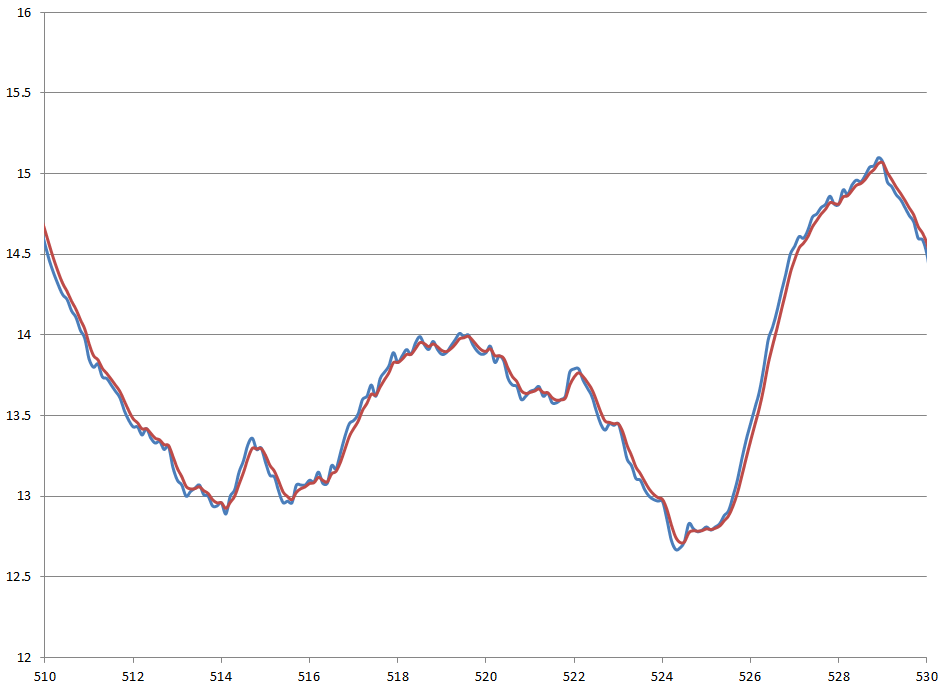下图为R取为0.5时的滤波效果，很明显，这张图信号的跟随效果比上图要差，滞后也多，但是滤波后曲线更平滑。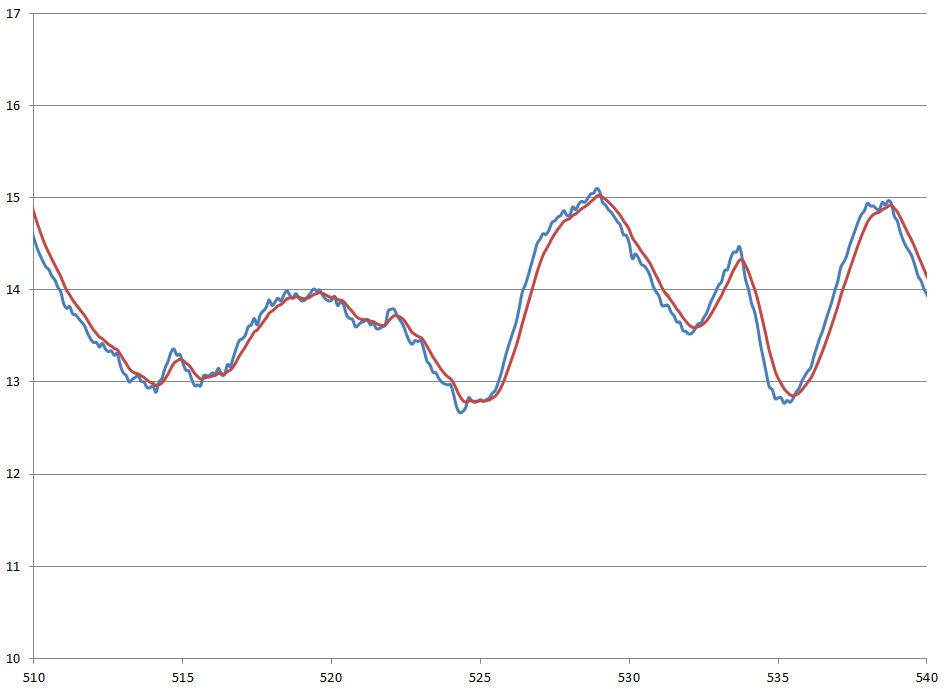展开全文无人驾驶 卡尔曼增益 一阶滤波
• 卡尔曼滤波算法从名称上来看落脚点是一个滤波算法，一般的滤波算法都是频域滤波，而卡尔曼滤波算法是一个时域滤波，这就是它的强大之处。再说说滤波这个名词，本质上就是给加权，其实深度学习中的卷积操作也是加权...

最近做卡尔曼滤波跟踪的项目，看原理花了一天，再网上查找并看懂别人的kalman c++代码花了我近三天的时间。卡尔曼滤波就是纸老虎，核心原理不难，核心公式就5个，2个状态预测更新公式，3个矫正公式。这里只讲解线性kalman滤波模型，非线性kalman滤波可以用扩散kalman滤波算法。

# 概述

卡尔曼滤波算法从名称上来看落脚点是一个滤波算法，一般的滤波算法都是频域滤波，而卡尔曼滤波算法是一个时域滤波，时域就是它的强大之处。卡尔曼滤波也是一种最优估计算法，常见的最优估计算法有“最小二乘法”等，卡尔曼滤波也是一个迭代器，根据以知的先验值，预测下一时刻的估计值。
再说说滤波这个名词，本质上就是给加权。既然是加权，那么卡尔曼滤波算法本质就是数据融合的操作(Data fusion)，卡尔曼增益就是融合过程中的权重，融合的内容有两个：分别是满足一定分布的先验状态估计值和满足一定分布的观测值之间的融合（也就是你们可能在其他资料上看到的两个椭圆相乘，其实就是数据的融合），融合后得到后验状态估计值。

其实深度学习中的卷积操作也是加权求和，本质上也是滤波，本质上也是求导，比如边缘检测的离散微分算子sobel的计算过程，也是分别在x和y两个方向上求导，提取出边缘特征。（下面是我个人的思考）由点及面，我们就可以想像到一个大尺寸的卷积核，其实就相当于“变形求导”，通过卷积核内部权重数值大小给定求导曲线，卷积就是求解在这条曲线上的导数。 一个卷积核是提取特征，那么多个卷积核组合起来就有无限可能，提取到的就是高维特征（高维特征对应图片的纹理等深层次的语义信息），组合起来非常之强大，就能够实现深度学习中的分类、边框回归等功能。

# 直接上菜 - 牛逼哄哄的五大公式下面来一个个详细剖析每个参数：
1.分别表示 k － 1 时刻和 k 时刻的后验状态估计值，是滤波的结果之一，即更新后的结果，也叫最优估计（估计的状态，根据理论，我们不可能知道每时刻状态的确切结果所以叫估计）。
2，: k 时刻的先验状态估计值，是滤波的中间计算结果，即根据上一时刻（k-1时刻）的最优估计预测的k时刻的结果，是预测方程的结果。
3，：分别表示 k － 1 时刻和 k 时刻的后验估计协方差（即的协方差，表示状态的不确定度），是滤波的结果之一。

4，：k 时刻的先验估计协方差（的协方差），是滤波的中间计算结果。

5，H：是状态变量到测量（观测）的转换矩阵，表示将状态和观测连接起来的关系，卡尔曼滤波里为线性关系，它负责将 m 维的测量值转换到 n 维，使之符合状态变量的数学形式，是滤波的前提条件之一。

6，：测量值（观测值），是滤波的输入。

7，：滤波增益矩阵，是滤波的中间计算结果，卡尔曼增益，或卡尔曼系数。

8，A：状态转移矩阵，实际上是对目标状态转换的一种猜想模型。例如在机动目标跟踪中， 状态转移矩阵常常用来对目标的运动建模，其模型可能为匀速直线运动或者匀加速运动。当状态转移矩阵不符合目标的状态转换模型时，滤波会很快发散。

9，Q：过程激励噪声协方差（系统过程的协方差）。该参数被用来表示状态转换矩阵与实际过程之间的误差。因为我们无法直接观测到过程信号， 所以 Q 的取值是很难确定的。是卡尔曼滤波器用于估计离散时间过程的状态变量，也叫预测模型本身带来的噪声。状态转移协方差矩阵

10：R: 测量噪声协方差。滤波器实际实现时，测量噪声协方差 R一般可以观测得到，是滤波器的已知条件。

11，B：是将输入转换为状态的矩阵

12，：实际观测和预测观测的残差，和卡尔曼增益一起修正先验（预测），得到后验

插曲一下：我认为最伟大的公式当属麦克斯韦方程组，十分的美妙，像一个妙龄少女，闭月羞花,沉鱼落雁，爱了爱了，哈哈哈。。。。
但是卡尔曼滤波也十分了不起，同样牛逼哄哄。
说到这儿，摆上大餐，提提文章档次，微分形式的麦克斯韦方程组（核心是两个散度两个旋度）：言归正传，转到正台：

# 建模（这里讲的数据都是矩阵）

卡尔曼滤波算法是需要建模的，拿高中物理中典型的例子举例，在二维平面中，跟踪一个质量为m的小球，t0时刻有水平速度v0，只受到重力作用运动，让你找出小球接下来的真实轨迹。你很快想到，很简单，我拿一个相机拍下小球的运动轨迹就好了。理论上没错，事实上拿相机记录也存在一定误差，怎么样来描述这个误差了，我们想到高斯噪声是最常见的噪声类型，那么我们假定相机的观测误差vk满足期望为‘0’协方差矩阵为R的高斯噪声vk~N(0,R)，协方差矩阵为R反应了测量噪声的分布情况,矩阵R的迹越大，说明误差越分散，进一步说明测量值置信度越低。
同时，我们建立一个理想模型来表述它或者叫预测它。假定系统的状态方程为(公式推导可以自己查阅资料)：这个状态方程是根据上一时刻的状态（这里指的是上一时刻的后验估计状态值，嗯，这样说比较准确）和控制变量来推测此刻的状态，由于世界上没有理想的理论模型，wk-1是服从高斯分布的噪声，是预测过程的噪声，它对应了 xk 中每个分量的噪声，期望为 0，协方差为 Q 的高斯白噪声wk-1~N(0,Q),Q即过程激励噪声协方差矩阵Q。

首先我们需要确定预测的状态变量数量和观测值，比如我们跟踪的是上面的模型，要知道小球的位置，那么我们可以给定预测4个状态值（x,y,vx,vy）,观测值就点的坐标（x,y）。我们可以通过k-1次的测量值，来估计第k次的先验估计值，再用卡尔曼增益值将第k次的测量值和第k次的先验估计值做融合，得到第k次的后验估计值，第k次的后验估计值就是我们求出的最优解。

# OpenCV中关于Kalman滤波的结构和函数定义

Kalman 滤波器状态，具体如何使用请查看opencv官方给的kalman案例。

typedef struct CvKalman
{
int MP;                     /* 测量向量维数 */
int DP;                     /* 状态向量维数 */
int CP;                     /* 控制向量维数 */

/* 向后兼容字段 */
#if 1
float* PosterState;         /* =state_pre->data.fl */
float* PriorState;          /* =state_post->data.fl */
float* DynamMatr;           /* =transition_matrix->data.fl */
float* MeasurementMatr;     /* =measurement_matrix->data.fl */
float* MNCovariance;        /* =measurement_noise_cov->data.fl */
float* PNCovariance;        /* =process_noise_cov->data.fl */
float* KalmGainMatr;        /* =gain->data.fl */
float* PriorErrorCovariance;/* =error_cov_pre->data.fl */
float* PosterErrorCovariance;/* =error_cov_post->data.fl */
float* Temp1;               /* temp1->data.fl */
float* Temp2;               /* temp2->data.fl */
#endif

CvMat* state_pre;           /* 预测状态 (x'(k)):
x(k)=A*x(k-1)+B*u(k) */
CvMat* state_post;          /* 矫正状态 (x(k)):
x(k)=x'(k)+K(k)*(z(k)-H*x'(k)) */
CvMat* transition_matrix;   /* 状态传递矩阵 state transition matrix (A) */
CvMat* control_matrix;      /* 控制矩阵 control matrix (B)
(如果没有控制，则不使用它)*/
CvMat* measurement_matrix;  /* 测量矩阵 measurement matrix (H) */
CvMat* process_noise_cov;   /* 过程噪声协方差矩阵
process noise covariance matrix (Q) */
CvMat* measurement_noise_cov; /* 测量噪声协方差矩阵
measurement noise covariance matrix (R) */
CvMat* error_cov_pre;       /* 先验误差计协方差矩阵
priori error estimate covariance matrix (P'(k)):
P'(k)=A*P(k-1)*At + Q)*/
CvMat* gain;                /* Kalman 增益矩阵 gain matrix (K(k)):
K(k)=P'(k)*Ht*inv(H*P'(k)*Ht+R)*/
CvMat* error_cov_post;      /* 后验错误估计协方差矩阵
posteriori error estimate covariance matrix (P(k)):
P(k)=(I-K(k)*H)*P'(k) */
CvMat* temp1;               /* 临时矩阵 temporary matrices */
CvMat* temp2;
CvMat* temp3;
CvMat* temp4;
CvMat* temp5;
}
CvKalman;


# 实践（C++）

目前经典的MOT多目标跟踪算法是sort、deepsort，其中sort算法是先经过Hungarian algorithm（匈牙利算法）进行最大匹配，再经过本文的kalman滤波进行预测。实际项目中我是先用常规（ssd或者yolo）等检测网络，检测出框，，再用最简单的IOU匹配跟踪，再用kalman进行预测。发现加了kalman滤波效果没提升，甚至框子抖动稍微大了一些（打印了kalman预测前后的数值，确认了kalman在工作）:原因可能是因为自己使用的是kalman均速模型，而我们神经网络检测出的物体框存在误差（抖动），所以如果每次检测框不准确（抖动过大或者忽大忽小），会导致均速的kalman模型预测也跟着抖动，甚至抖动幅度更大了。切换到加速度kalman模型，发现乱框，加速度kalman模型不能用在目标检测框跟踪上。项目上参考的代码是：https://github.com/Smorodov/Multitarget-tracker
由于kalman滤波需要建模，所以它适用于跟踪目标符合一定的规律或者趋势，检测框的不确定性太大了，可以试试，一般效果不大，最终还是取决于检测效果的好坏。

最后收尾：每一个物理学家的必定都是数学家。

展开全文• 代码的集合，这些代码是作为课程参与的一部分编写的并获得证书。到目前为止，这是我参加过的最苛刻的课程之一，需要 97% 的及格率才能获得证书。然而，fru 它的辛勤劳动很棒，并决定与社区的其他人分享。
• 试利用扩展卡尔曼滤波理论求出的最优估计。 要求： （1）利用Matlab或Python 编写仿真程序。 （2）给出各状态变量的真值和估计值曲线变化图。 （3）分别给出的真值与估计值之间的误差曲线变化图，并求出误差的均值和...
• ## 卡尔曼滤波算法及其python实现

万次阅读 多人点赞 2020-01-07 15:20:54
卡尔曼滤波算法及其python实现算法原理python实现 算法原理 python实现 # KF algorith demo by Leo # 2020.01.06 # ZJG CAMPUS,ZJU import numpy as np import matplotlib.pyplot as plt ''' 生成带噪声的...

# 算法原理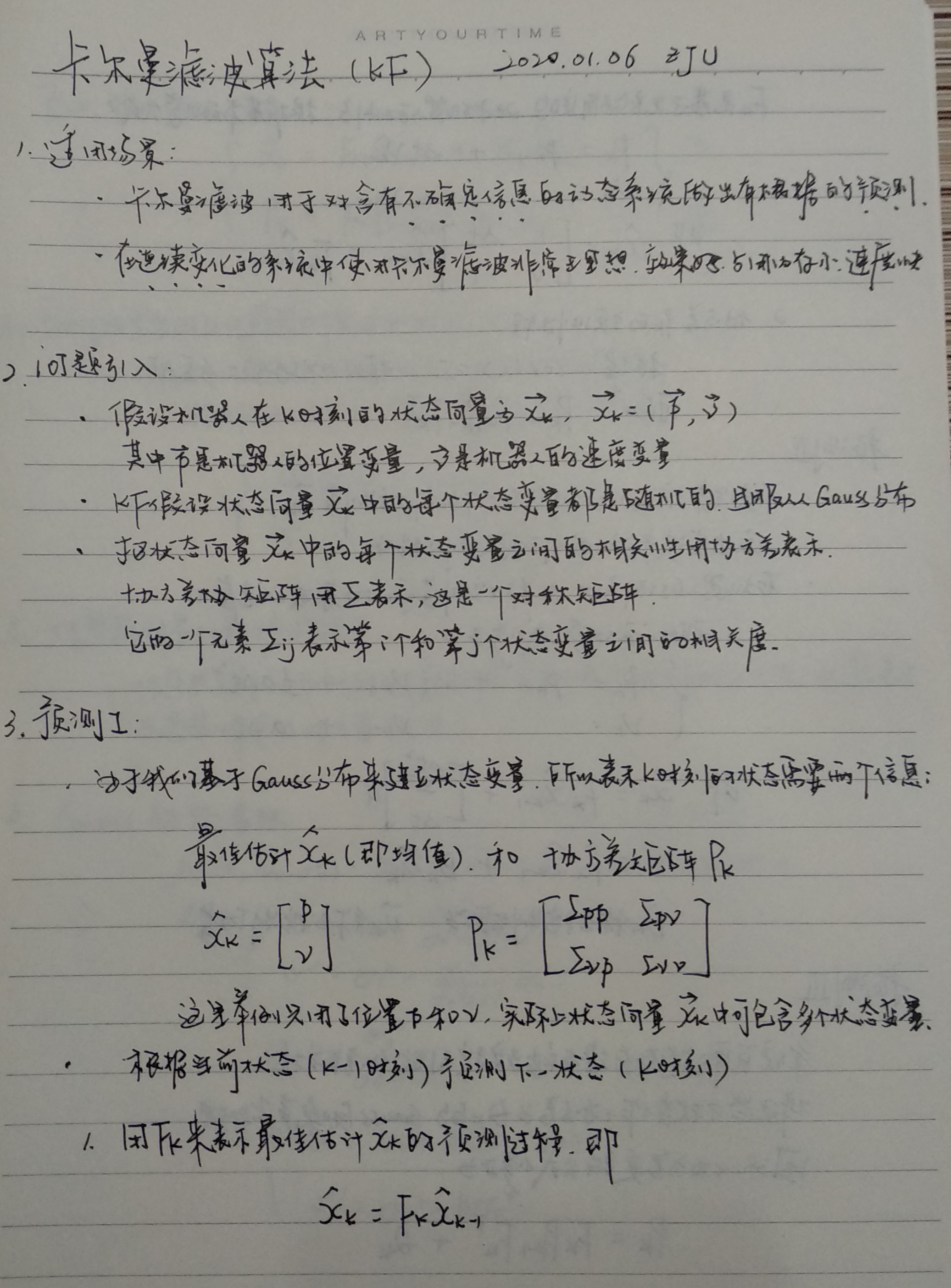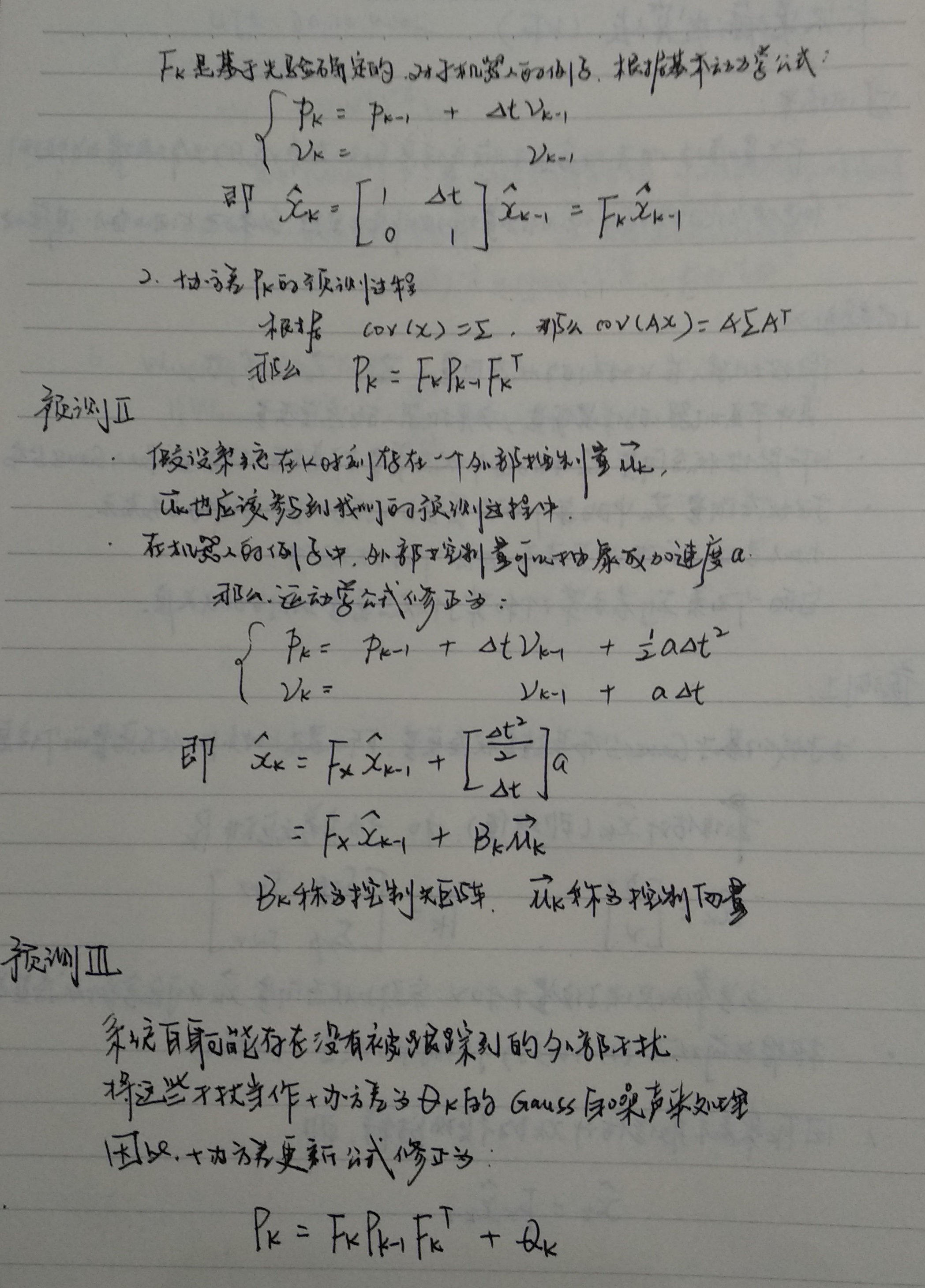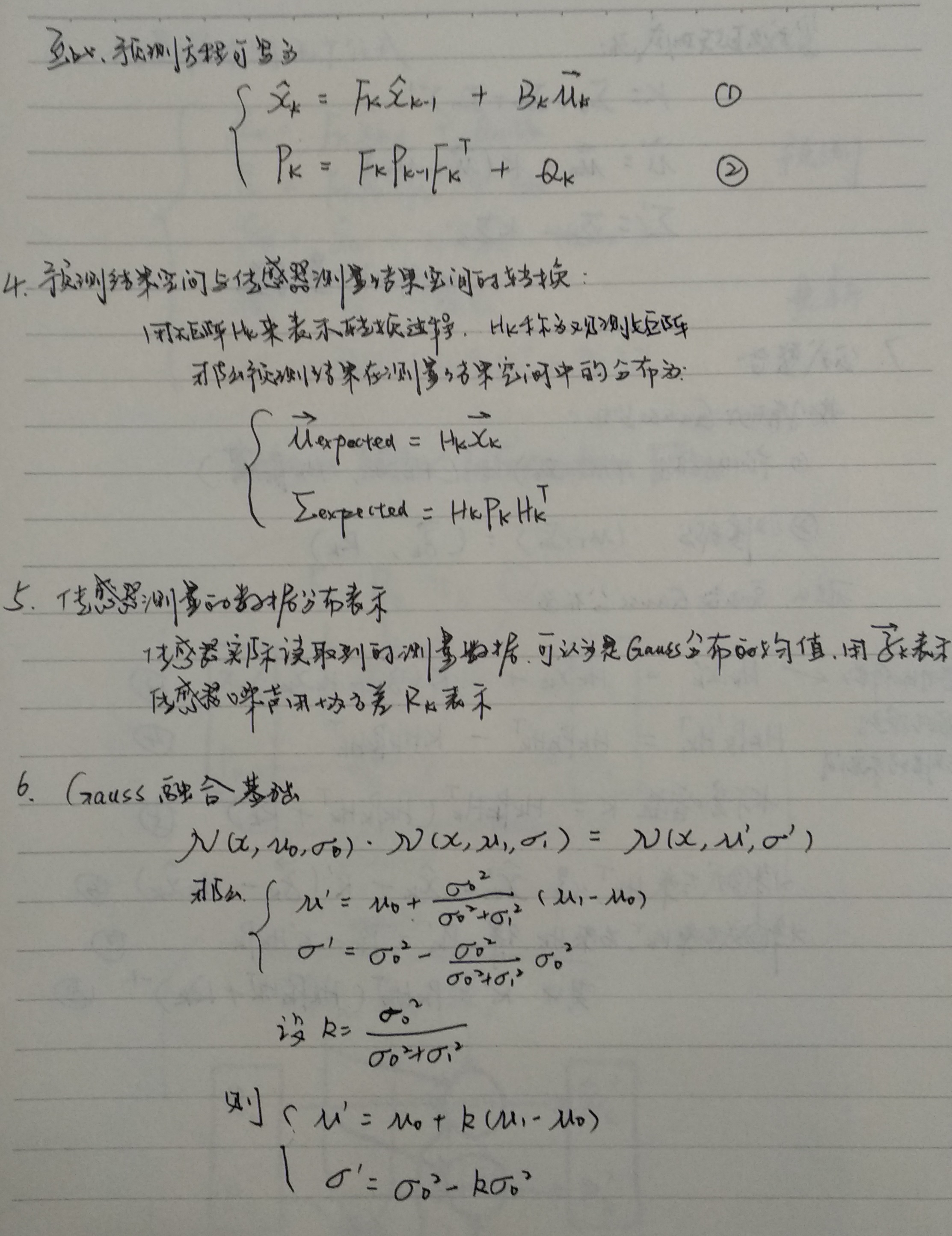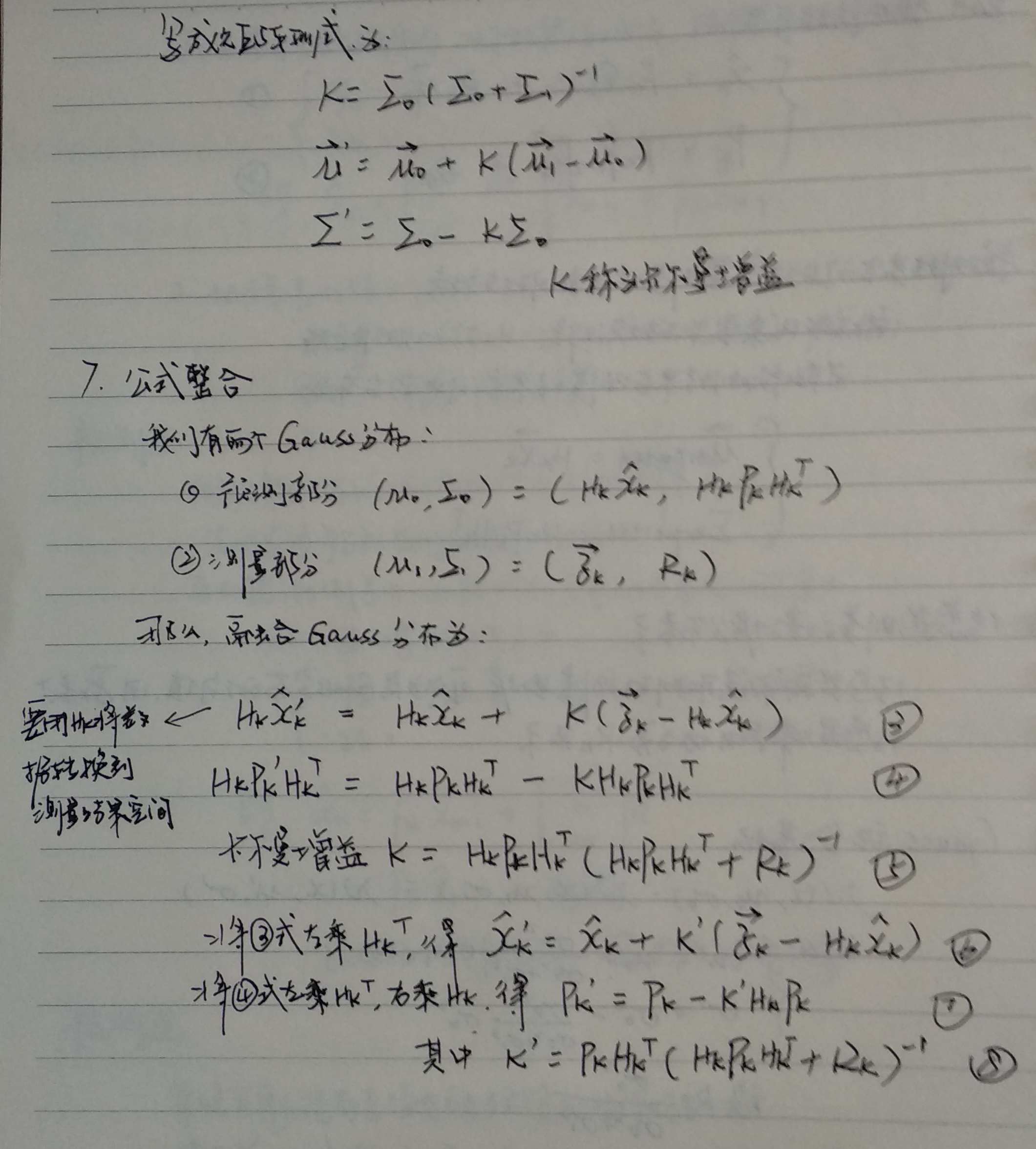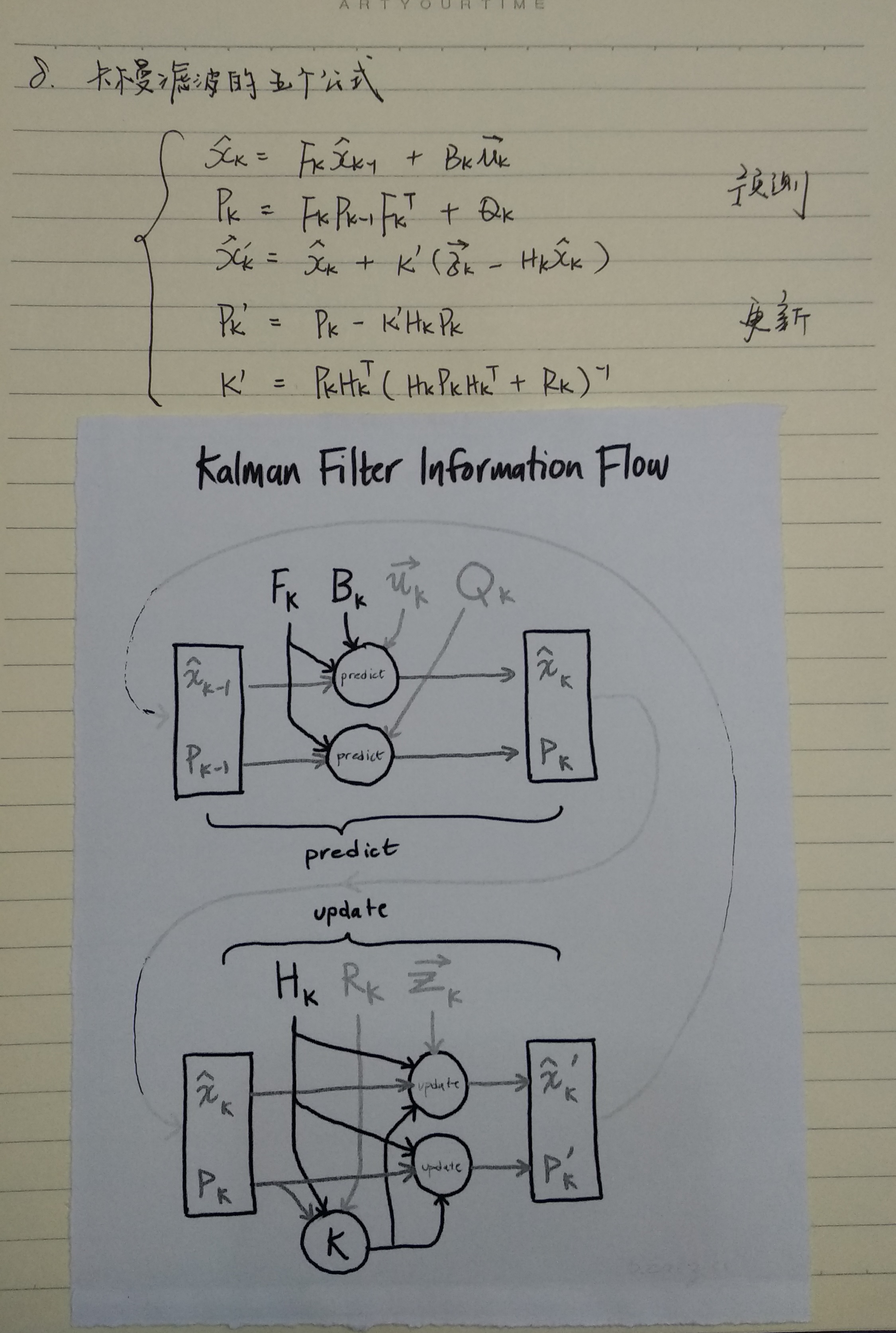# python实现

# KF algorith demo by Leo
# 2020.01.06
# ZJG CAMPUS,ZJU

import numpy as np
import matplotlib.pyplot as plt

'''
生成带噪声的传感器观测值Z
Z中一共包含500个samples，第k个sample代表k时刻传感器的读数
假设只对机器人位置进行传感器观测，并且只用距离表示位置
因此，Z中只有一个观测变量，即机器人的位置，这个位置一维数据表示
'''
# 生成不带噪声的数据
Z_raw = [i for i in range(500)]
# 创建一个均值为0，方差为1的高斯噪声，共有500个samples，精确到小数点后两位
noise = np.round(np.random.normal(0, 1, 500), 2)
# 将z的观测值和噪声相加
Z = np.mat(Z_raw) + np.mat(noise)

'''
定义状态向量X的初始状态
X中包含两个状态变量：p和v，二者都被初始化为0，且二者都用标量表示
'''
X = np.mat([[0,], [0,]])

'''
定义初始状态协方差矩阵P
'''
P = np.mat([[1, 0], [0, 1]])

'''
定义状态转移矩阵F，假设每秒钟采一次样，所以delta_t = 1
'''
F = np.mat([[1, 1], [0, 1]])

'''
定义状态转移协方差矩阵Q
这里我们把协方差设置的很小，因为觉得状态转移矩阵准确度高
'''
Q = np.mat([[0.0001, 0], [0, 0.0001]])

'''
定义观测矩阵H
'''
H = np.mat([1, 0])

'''
定义观测噪声协方差R
'''
R = np.mat()

'''
卡尔曼滤波算法的预测和更新过程
'''
for i in range(100):
x_predict = F * X#demo中没有引入控制矩阵B
p_predict = F * P * F.T + Q
K = p_predict * H.T / (H * p_predict * H.T + R)
X = x_predict + K *(Z[0, i] - H * x_predict)
P = (np.eye(2) - K * H) * p_predict
print(X)
plt.plot(X[0, 0], X[1, 0], 'ro', markersize = 4)

plt.show()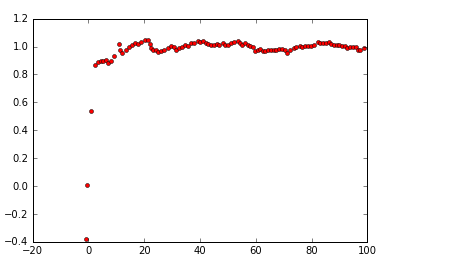其中，横轴表示X[0,0]，即位置p; 纵轴表示X[1,0]，即速度v
可以看到速度v很快收敛于1.0，这是因为设置delta_t=1，即Z中的数据从0-500，每秒加1，卡尔曼滤波预测的速度与实际速度1.0很好的契合。
并且，我相信如果将横轴展开来看，卡尔曼滤波也对位置的预测具有很好的契合。

# 参考资料

1.[blog]详解卡尔曼滤波原理
翻译自http://www.bzarg.com/p/how-a-kalman-filter-works-in-pictures/
blog地址：https://blog.csdn.net/u010720661/article/details/63253509
2.[blog]我所理解的卡尔曼滤波
blog地址：https://www.jianshu.com/p/d3b1c3d307e0
3.[blog]卡尔曼滤波,最最容易理解的讲解.找遍网上就这篇看懂了.
blog地址：https://blog.csdn.net/phker/article/details/48468591
4.[paper]A New Approach to Linear Filtering
and Prediction Problems
paper地址：http://www.cs.unc.edu/~welch/kalman/media/pdf/Kalman1960.pdf

展开全文原理 python实现
• ## 卡尔曼滤波算法matlab实现

千次阅读 多人点赞 2021-07-23 13:31:48
卡尔曼滤波方程 简单示例程序 %% 参数设置 N = 200; % 设置数据长度为N t = (1:N); % 生成时间轴 a = 1; % 状态转移方程 b = 0; % 控制输入 c = 1; % c: 观测方程 x = 5; % 设置初值 初始的均值和方差 sigma2 = ...
• 用Fortran编写的kalman滤波模拟算法，包含源程序和数据
• 卡尔曼滤波（平方根算法、序贯算法、线性卡尔曼滤波、扩展卡尔曼滤波、区间卡尔曼滤波、系统误差和观测误差有关的卡尔曼滤波）在R语言中的实现，详细讲述了原理和其中工具包的使用，英文原版。
• 基于改进迭代扩展卡尔曼滤波的3星时频差测向融合动目标跟踪方法[J]. 电子与信息学报, 2021, 43(10):7. 博主简介：擅长智能优化算法、神经网络预测、信号处理、元胞自动机、图像处理、路径规划、无人机等多种领域的...目标跟踪 matlab
• ## 利用Python实现卡尔曼滤波算法

万次阅读 多人点赞 2018-09-08 17:04:41
绿线为原值，黄线为滤波后的数据，蓝线为加入噪声的数据。 3、改进版## import numpy as np import matplotlib . pyplot as plt class kalman_filter : def __init__ ( self , Q , R ) : ...ADC python
• 大数据-算法-基于集合卡尔曼滤波的遥感信息和作物模型结合研究.pdf
• 大数据-算法-基于集合卡尔曼滤波法的非高斯含水层参数识别.pdf
• 首先明确一下卡尔曼滤波的基本概念：可参考知乎诸位大神的“如何通俗易懂地描述卡尔曼滤波“，这里我也稍微说明一下。 所谓卡尔曼滤波就是当你在测量一个值时，同时拥有模型估计和直接测量两种方式，但是两种方式都...
• 卡尔曼滤波是一种高效率的递归滤波器（自回归滤波器），它能够从一系列的不完全及包含噪声的测量中，估计动态系统的状态。 卡尔曼滤波的一个典型实例是从一组有限的，包含噪声的，通过对物体位置的观察序列...
• 无损卡尔曼滤波又称无迹卡尔曼滤波（Unscented Kalman Filter，UKF），是无损变换（Unscented Transform，UT）与标准卡尔曼滤波体系的结合，通过UT变换使非线性系统方程适用于线性假设下的标准卡尔曼体系。...
• 详解神奇的卡尔曼滤波（Kalman filter）算法 文章目录 详解神奇的卡尔曼滤波（Kalman filter）算法 前言 一、卡尔曼滤波要解决的问题 二、贝叶斯滤波 三、卡尔曼滤波的详细推导 四、扩展卡尔曼滤波（EKF） 前言 任何...矩阵
• 大数据-算法-四维变分和集合卡尔曼滤波同化多普省略方法及其反演暴雨中尺度结构的研
• 1．简介(Brief Introduction)在学习卡尔曼滤波器之前，首先看看为什么叫“卡尔曼”。跟其他著名的理论（例如傅立叶变换，泰勒级数等等）一样，卡尔曼也是一个人的名字，而跟他们不同的是，他是个现代人！卡尔曼全名...
• 所有滤波问题其实都是求感兴趣的状态的后验概率分布，只是由于针对特定条件的不同，可通过求解析解获得后验概率（KF、EKF、UKF），也可通过大数统计平均求期望的方法来获得后验概率（粒子滤波）。 KF、EKF、UKF ...
• 大数据-算法-基于集合卡尔曼滤波的遥感信息和作物模型结合研究——以东北地区玉米估产
• # gain or blending factor卡尔曼增益 R = 0.1**2 # estimate of measurement variance, change to see effect intial guesses xhat = 0.0 P = 1.0 for k in range(1,num_iter): # 预测 xhatminus[k] = xhat[k...python...